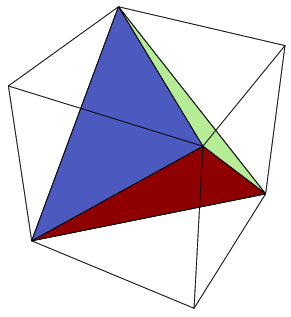# A Geometric Higher Dimensional Phenomenon?

Geometry Level 3In $\text{3D}$ it's possible to make a regular tetrahedron with integer coordinates that all lie on the vertices of a cube.

Does this phenomenon occur in any other dimensional space?

Or specifically, for how many $n \in \mathbb{N}$ is it possible to construct a regular $n$-dimensional simplex with integer co-ordinates that lie on an $n$-dimensional hypercube in $\mathbb{Z}^n$?

Details and Assumptions:

• A regular $n$-dimensional simplex in $\mathbb{Z}^n$ has $n+1$ vertices that are all an equal distance apart. (It's like an $n$-dimensional version of an equilateral triangle!)

• Here is the Wikipedia article on hypercubes. (It's like an $n$-dimensional version of a square!)

• Note that the analogous phenomena is not possible in $2$ dimensions. That is, we cannot create an equilateral triangle with integer co-ordinates that all lie on a square. Check out why, here.

×

Problem Loading...

Note Loading...

Set Loading...